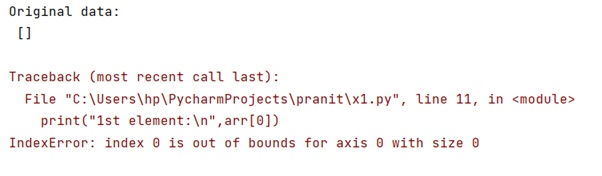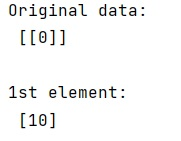# What does 'index 0 is out of bounds for axis 0 with size 0' mean in Python?

Learn about the 'IndexError: index 0 is out of bounds for axis 0 with size 0', and how to fix it? By Pranit Sharma Last updated : April 03, 2023

## IndexError: Index 0 is out of bounds for axis 0 with size 0

While working with numpy arrays, we often use indexing with arrays and in the case of 2D arrays, there are multiple rows that we use in indexing.

Index and dimension numbering starts with 0. So, axis 0 means the 1st dimension. Also, in numpy, a dimension can have a length (size) of 0.

The simplest example can be given as:

### Example

```# Import numpy
import numpy as np

# Creating a numpy array using random values
arr = np.zeros((0,), int)

# Display original data
print("Original data:\n",arr,"\n")

# Indexing
print("1st element:\n",arr)
```

### Output## How to fix 'IndexError: Index 0 is out of bounds for axis 0 with size 0'?

We get this error as our array has no dimensions and does not have any element at the 0th index. To fix index 0 is out of bounds for axis 0 with size 0, add a dimension and place some element at this position, it will print that element.

### Solution

```# Import numpy
import numpy as np

# Creating a numpy array using random values
arr = np.zeros((1,1), int)

# Display original data
print("Original data:\n",arr,"\n")

arr = 10

# Indexing
print("1st element:\n",arr)
```

### Output